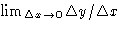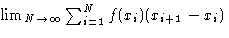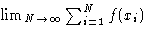Up: Subject of Statistical Thermodynamics Previous: Phase Space

# Quiz

This quiz is not graded. Check all applicable answers for multiple choice questions.
1.
What is a derivative of a function?
(a)
Difference of maximal and minimal values of the function
(b)
Slope of the tangent at a given point
(c)(d)
Speed of function's change
(e)
The lowest value of a function
2.
What is an integral?
(a)
Area under graphics of the function
(b)
Sum of the minimal and maximal value of the function
(c)(d)(e)
The largest value of the function
3.
Area of a square with side a is a2. Volume of a cube with the side a is a3. What is the volume of an N-dimensional hypercube with the side a?
4.
What is the probability to have a sum of three when throwing two dice? A sum of four?
5.
What is the dimension of the phase space for a system, that has only one particle moving along a straight line? Draw a trajectory in a phase space for a particle that has a constant velocity.
6.
What is the dimension for the phase space for a harmonic oscillator? Sketch its trajectory in the phase space.Up: Subject of Statistical Thermodynamics Previous: Phase Space

© 1997 Boris Veytsman and Michael Kotelyanskii
Wed Sep 3 22:38:51 EDT 1997